### Introduction

Personal collection of theorems, lemmas, notes, and diagrams fundamental to the control literature of the past seventy-some years. I keep updating this post time after time. Mostly deals with nonlinear control, as the world is nonlinear.

### Feedback Linearization

What follows closely follows Sastry and Bodson’s Adaptive Control: Stabilty, Convergence, and Robustness definitions; as well as Ioannis’s Adaptive Control Tutorial book (I noticed a few mistakes in the version of his book that I have, in Chapter 9 to be exact, and I’ve tried to state definitions as correctly as my senses can make them here).

Feedback linearization consists in changing the coordinates of a system so as to cancel all or most of the unknown nonlinear terms so that the system behaves as a linear or partly linear system. Consider the following system

\begin{align} \dot{x} = f(x) + g(x)u, \nonumber \end{align}

\begin{align} y = h(x) \label{eq:nlnr1} \end{align}

where $x \in \mathbb{R}^n, u,y \in \mathbb{R} \text{ and } f,g,h,$ are smooth nonlinear functions. Differentiating $y$ in \eqref{eq:nlnr1} with respect to time, we find that

\begin{align} \dot{y} = \dfrac{\partial{h}}{\partial{x}}(x) f(x) + \dfrac{\partial{h}}{\partial{x}}(x) g(x) u, \label{eq:ydot} \end{align}

Define \begin{align} L_f \, h \triangleq \dfrac{\partial{h}}{\partial{x}}(x) f = \dfrac{\partial{h}}{\partial{x}_1}(x) f_1 + \cdots + \dfrac{\partial{h}}{\partial{x}_n}(x) f_n, \label{eq:Lie} \end{align}

called the Lie derivative of $h(x)$ w.r.t $f(x)$, so that we can rewrite \eqref{eq:ydot} as

\begin{align} \dot{y} \triangleq L_f \, h + L_g h(x) u. \label{eq:ydot_lie} \end{align}

If $\frac{\partial{h}}{\partial{x}}(x_0) \, g(x_0) \neq 0$ (i.e. $L_g h(x_0) \neq 0$) at some point $x_0$, then the system \eqref{eq:nlnr1} is said to be of relative degree 1 at $x_0$. For an LTI system, this means the output is different from the input by one integrator only. This would be a strictly proper tranfer function. A good way of thinking about this is that the output has to be differentiated by the number of the relative degree(s) until the input appears in the output expression. If $L_g h(x)$ is bounded away from $0$ for all $x$, the feedback control law can be written as

\begin{align} u = \dfrac{1}{L_g}h(-L_f h + \nu) \end{align}

allowing us to write a linear system from $\nu$ to output $y$ as

\begin{align} \dot{y} = \nu \end{align}

which can render the $(n-1)$ states of \eqref{eq:nlnr1} unobservable by a feedback choice.

If $L_g h(x) = 0 \, \forall \, x \in {B_x}_0 \text{ in some neighborhood } {B_x}_0$, then one can take the second derivative of $y$ to obtain (abusing notation and dropping the terms in parentheses),

\begin{align} \ddot{y} = \dfrac{\partial}{\partial{x}} \left(\dfrac{\partial{h}}{\partial{x}}f\right)f + \dfrac{\partial}{\partial{x}}\left(\dfrac{\partial{h}}{\partial{x}}f\right) gu \triangleq L_f^2 h(x) + (L_g L_fh)(x)u. \end{align}

If $\frac{\partial}{\partial{x}} \left(\frac{\partial{h}}{\partial{x}}(x)f(x)\right) g(x) \, |\ _{x = x_0} \neq 0$ then \eqref{eq:nlnr1} is said to have a relative degree 2 at $x_0$. We can continue the differentiation in a neighborhood of $x_0$ if $\frac{\partial}{\partial{x}} \left(\frac{\partial{h}}{\partial{x}}(x)f(x)\right)g(x) = 0$ in the neighborhood of $x_0$.

There are two general cases of adopting adaptive control to nonlinear systems: (i) nonlinear systems whose nonlinear functions are known but unknown parameters appear linearly. (ii) the nonlinear functions are assumed known by multiplying nonlinear basis functions with unknown parameters to be determined.

More generally,

\begin{align} L_f^i h = \dfrac{\partial}{\partial{x}}\left(\dfrac{\partial}{\partial{x}} \left(\cdot \cdot \cdot \dfrac{\partial}{\partial{x}} \left(\dfrac{\partial{h}}{\partial{x}}f\right) \cdot f \cdots \right) \cdot f \right) \cdot f. \end{align} where the partial derivatives of the r.h.s are carried out $i$ times. Let

\begin{align} L_g L_fh \triangleq \frac{\partial(L_fh)}{\partial x}\cdot g, \end{align}

then the nonlinear SISO system \eqref{eq:nlnr1} has a relative degree $\rho = n$ at a point $x_0$ if

(i) $L_g L_f^i h(x) = 0 \forall x \in {B_x}_0,$ where ${B_x}_0$ is some neighborhood of $x_0$ $\forall i = 1, 2, 3, \ldots, \rho - 2.$

(ii) $L_g L_f^{\rho -1} h(x_0) \neq 0.$

if \eqref{eq:nlnr1} has relative degree $\rho = n$ at $x$, where $n$ is the order of \eqref{eq:nlnr1}, then given the transfoirmation

\begin{align} z_1 = y = h(x), \qquad z_2 = \dot{y} = L_f h(x), \qquad z_3 = \ddot{y} = L_f^2 h(x), \end{align}

\begin{align} z_i = y^{(i-1)} = L_f^{(i-1)} h(x) , \ldots, z_n = y^{(n-1)} = L_f^{n-1} h(x). \end{align}

we find that

$\dot{z}_1 = z_2, \nonumber \\ \dot{z}_2 = z_3, \nonumber \\ \quad \vdots \nonumber \\ \dot{z}_{n-1} = z_n, \nonumber \\ \dot{z}_n = L_f^nh(x) + (L_gL_f^{n-1}h(x))u, \\ y = z_1 \label{eq:canon}$

which is the canonical form of the system with no zero dynamics. From feedback linearization, we find that

\begin{align} u = \dfrac{1}{L_g L_f^{n-1}h(x)}[\nu - L_f^n h(x)], \label{eq:control} \end{align}

where $\nu \in \mathbb{R}$ is the new input, leading to the LTI system

$\dot{z}_1 = z_2, \\ \dot{z}_2 = z_3, \\ \quad \vdots \\ \dot{z}_{n-1} = z_n, \\ \dot{z}_n = \nu, \\ y = z_1$

Vectorizing the two equations, we have

\begin{align} \dot{z} = A z + B \nu, \qquad y = C^T z, \label{eq:linear} \end{align}

where

$A = \begin{bmatrix} 0 & 1 & 0 & \ldots & 0 \\ \vdots & \ddots & \ddots & \ddots & \vdots \\ \vdots & & \ddots & \ddots & 0 \\ \vdots & & & \ddots & 1 \\ 0 & \ldots & \ldots & \ldots & 0 \\ \end{bmatrix}, \qquad B = \begin{bmatrix} 0 \\ \vdots \\ \vdots \\ 1 \end{bmatrix}, \quad C = \begin{bmatrix} 1 \\ 0 \\ \vdots \\ \vdots \\ 0 \end{bmatrix}$

\eqref{eq:linear} is an observable and controllable LTI system, and thus the input $\nu$ can be carefully chosen to meet regulation or tracking objectives for the plant output $y$. The \eqref{eq:control} cancels all nonlinearities and turns the closed-loop system to an LTI one. If \eqref{eq:nlnr1} has relative degree $\rho < n$, the change of coordinates become

\begin{align} z_1 = y, \quad z_2 = \dot{y}, \ldots \quad z_\rho = y^{(\rho-1)} = L_f^{(\rho -1)h(x) } \end{align}

thus resulting in

$\dot{z}_1 = z_2 \\ \vdots \\ \dot{z}_{\rho-1} = z_\rho \\ \dot{z}_\rho = L_f^\rho h(x) + \left(L_gL_f^{\rho-1}h(x)\right) u$

Since the order of the system is $n$, we are gonna need $n - \rho$ states; we can define functions $h_{\rho+1}(x), \ldots, h_n(x)$ with $\frac{\partial{h}_i(x)}{\partial{x}g(x)} = 0, \, i = \rho +1, \ldots, n$ and define the $n - \rho$ states as

$z_{\rho+1} = h_{\rho + 1}, \ldots, z_n = h_n(x)$

$\dot{z}_{\rho+1} = \dfrac{\partial{h}_{\rho+1}(x)}{\partial{x}}\cdot f(x) \triangleq \phi\_{\rho +1}(z), \\ \quad \vdots \\ \dot{z}_{n} = \dfrac{\partial{h}_{n}(x)}{\partial{x}}\cdot f(x) \triangleq \phi_{n}(z), \\ y = z_1$

where $z = [z_1, z_2, \ldots, z_n]^T$ is the new state. With feedback linearization, we have

\begin{align} u = \dfrac{1}{L_g L_f^{\rho-1}h(x)}[\nu - L_f^\rho h(x)], \label{eq:control2} \end{align}

so that we have the system

$\dot{z}_1 = z_2 \\ \vdots \\ \dot{z}_{\rho-1} = z_\rho \\ \dot{z}_\rho = \nu, \\ \dot{\rho + 1} = \phi_{\rho +1}(z), \\ \quad \vdots \\ \dot{z}_n = \phi_n(z), \\ y = z_1.$

We see that the input $\nu$ may be utilized to drive the output $y$ and states $z_1, \ldots, z_\rho$ to zero or meet some regulation goal for $y$. When the choice of controller $\nu$ does not guarantee that the states $z_{\rho+1}, \ldots, z_n$ are bounded despite $z_1, \ldots, z_\rho$ being driven to zero, we say the states $\dot{z}_{\rho+1}, \ldots, \dot{z}_n$ are the zero dynamics of \eqref{eq:nlnr1}. These are the dynamics of \eqref{eq:nlnr1} when $y$ and its first $\rho$ derivatives are set to zero. When the equilibrium states of the $z_{\rho+1}$ are asymptotically stable, the system is said to be minimum-phase.

The process illustrated above is called I/O feedback linearization. When there are no zero dynamics involved, the process is called full-state feedback linearization.

## Control Lyapunov Functions

Lyapunov functions are useful not only in the analysis of adaptive control systems but also as stabilizing feedback control design laws. The central concept is to make the Lyapunov function $V$ and its time derivative obey Lyapunov stability conditions for a closed-loop system.

### Lyapunov’s Direct Method

Lyapunov stability means the sum of all the energy of a system will be continuously dissipated when the system settles to an equilibrium position. This is a statement of the Lyapunov’s Direct (or second) method. In other words, we can investigate the stability of an $n$-dimensional dynamical system by analyzing the change in the behavior of its energy function. Stability analyses are difficult to verify in nonlinear systems and Lyapunov analysis helps us in gaining an insight into system behavior. Take a mass-spring-damper system below, for example.Pulling and releasing the mass does not inform us of the system’s stability whatsoever. The question of stability in the system is therefore difficult to verify:4, linearization is nearly impossible as it is only marginally stable. But if we can write out the closed-form equations of the system’s mechanical energy, we can differentiate this to understand what happens if the mass settles to equilibrium. For observe,

\begin{align} V(x) &= \underbrace{\dfrac{1}{2}m \dot{x}^2}_{\text{kinetic energy}} + \underbrace{\int_{0}^{x}\left(k_0x + k_1x^3\right)dx}_{\text{potential energy}} \end{align}

\begin{align} &= \frac{1}{2}m \dot{x}^2 + \dfrac{1}{2}k_0x^2 + \dfrac{k_1}{4} x^4 \end{align}

The rate of change of the system’s energy along the trajectories of the system’s motion therefore becomes

\begin{align} \dot{V}(x) &= m \dot{x} \ddot{x} + \left(k_0 x + k_1x^3\right) \dot{x} \end{align}

\begin{align} &= \dot{x} \left(-b \dot{x} |\dot{x}|\right) = -b |\dot{x}|^3 \le 0 \end{align}

#### Nonlinear Control Theory

• A differential equation of the form

\begin{align} dx/dt = f(x, u(t), t), \quad -\infty < t < +\infty \label{eq:diff_eq} \end{align}

is said to be free (or unforced) if $u(t) \equiv 0$ for all $t$. That is \eqref{eq:diff_eq} becomes

\begin{align} dx/dt = f(x, t), \quad -\infty < t < +\infty \label{eq:unforced} \end{align}

• If the differential equation in \eqref{eq:diff_eq} does not have an explicit dependence on time, but has an implicit dependence on time, through $u(t)$, then the system is said to be stationary. In other words, a dynamic system is stationary if

\begin{align} f(x, u(t), t) \equiv f(x, u(t)) \label{eq:stationary} \end{align}

• A stationary system \eqref{eq:stationary} that is free is said to be invariant under time translation, i.e.

\begin{align} \Phi(t; x_0, t_0) = \Phi(t + \tau; x_0, t_0 + \tau) \label{eq:free_stat} \end{align} - $\Phi(t; x_0, t_0)$ is the analytical solution to \eqref{eq:diff_eq}; it is generally interpreted as the solution of \eqref{eq:diff_eq}, with fixed $u(t)$, going through state $x_0$ at time $t_0$ and observed at time $t$ later on. This is a clearer way of representing the d.e.’s solution as against $x(t)$, which is popularly used in most text nowadays.

• $\Phi(\cdot)$ is generally referred to the transition function, since it relates the transformation from $x(t_0)$ to $x(t)$.

• For a physical system, $\Phi$ has to be continuous in all of its arguments. .

• If the rate of change $dE(x)/dx$ of an isolated physical system is negative for every possible state x, except for a single equilibrium state $x_e$, then the energy will continually decrease until it finally assumes its minimum value $E(x)$.

• The first method of Lyapunov deals with questions of stability using an explicit representation of the solutions of a differential equation
• Note that the second method is more of a historical misnomer, perhaps more accurately described as a philosophical point of view rather than a systematic method. Successful application requires the user’s ingenuity.
• In contrast to popular belief that the energy of a system and a Lyapunov function are the same, they are not the same. Why? Because the Lyapunov function, $V(x)$, is not unique. To quote Kalman, “a system whose energy $E$ decreases on the average, but not necessarily at each instant, is stable but $E$ is not necessarily a Lyapunov function.”

• Lyapunov analysis and optimization: Suppose a performance index is defined to be the error criterion between a measured and an estimated signal; suppose further that this criterion is integrated w.r.t time, then the performance index is actually a Lyapunov function – provided that the error is not identically zero along any trajectory of the system.

• Existence, uniqueness, and continuity theorem:

Let $f(x, t)$ be continuous in $x,t$, and satisfy a Lipschitz condition in some region about any state $x_0$ passing through time $t_0$:

\begin{align} R(x_0, t_0) &= ||x - x_0|| \le b(x_0) \nonumber \end{align}

\begin{align} R(x_0, t_0) &= ||t - t_0|| \le c(t_0) \quad (b, c) > 0 \end{align}

with the Lipschitz condition satisfied for $(x,t), (y,t)$ $\in$ $R(x_0, t_0)$, then it follows that \begin{align} ||f(x,t) - f(y,t)|| \le k \, ||x-y|| \nonumber \end{align}

where $k>0$ depends on $b, c$. THUS,

• there exists a unique solution $\Phi(t; x_0, t_0)$ of $dx/dt$, that starts as $x_0, t_0$ for all $|t - t_0| \le a(t_0)$,

• $a(t_0) \ge \text{ Min (}{c(t_0), b(x(t_0))/M(x_0, t_0)}$, where $M(x_0, t_0)$ is the maximum assumed by the continuous function $f(x,t)$ in the closed, bounded set $R(x_0, t_0)$

• in some small neighborhood of $x_0, t_0$, the solution is continuous in its arguments

Observe that the Lipschitz condition only implies continuity of $f$ in $x$ but not necessarily in $t$; as it is implied by the bounded derivatives in $x$. Note that the local lipschitz condition required by the theorem only implies desired properties of a solution near $x_0, t_0$.

The finite escape time (that is the solution leaves any compact set within a finite time) quandary does not allow us to make conclusions surrounding arbitrarily large values of $t$. The phrase “finite escape time” describes the concept that a trajectory escapes to infinity at a finite time. In order that a differential equation accurately represent a physical system, the possibility of finite escape time has to be mitigated by an explicit assumption to the contrary. If the Lipschitz condition holds for $f$ everywhere, then there can be no finite escape time. The proof is easy by integrating both sides of \eqref{eq:diff_eq} and using

\begin{align} \Phi(t; x_0, t_0) \le ||x_0|| + || \int_{t_0}^{t}f(\Phi(\tau; x_0, t_0), \tau)d\tau || \end{align}

\begin{align} ||x_0|| + k \int_{t_0}^{t}f(\Phi(\tau; x_0, t_0), \tau)d\tau \end{align}

where $f(\cdot)$ obeys the lipschitz condition,

\begin{align} ||f(x,t) - f(y,t)|| \le k \, ||x-y||. \nonumber \end{align}

By the Gronwall-Bellman lemma,

\begin{align} ||\Phi(t; x_0, t_0) || \le [\exp \, k (t - t_0)] ||x_0 || \nonumber \end{align}

which is less than $\infty$ for any finite $(t - t_0)$.

### Stability

My definitions follow from R.E Kalman’s 1960 seminal paper since they are clearer to understand compared to the myriad of definitions that exist in many texts today. Stability concerns the deviation about some fixed motion. So, we will be considering the deviations from the equilibrium state $x_e$ of a free dynamic system.

Simply put, here is how Kalman defines stability, if \eqref{eq:diff_eq} is slightly perturbed from its equilibrium state at the origin, all subsequent motions remain in a correspondingly small neighborhood of the origin. Harmonic oscillators are a good example of this kind of stability. Lyapunov himself defines stability like so:

• An equilibrium state $x_e$ of a free dynamic system is stable i.e. for every real number $\epsilon>0$, there exists a real number $\delta(\epsilon, t_0)>0$ such that $|x_0 - x_e| \le \delta$ implies

\begin{align} ||\Phi(t; x_0, t_0) - x_e|| \le \epsilon \quad \forall \quad t \ge t_0 \nonumber \end{align}

This is best imagined from the figure below: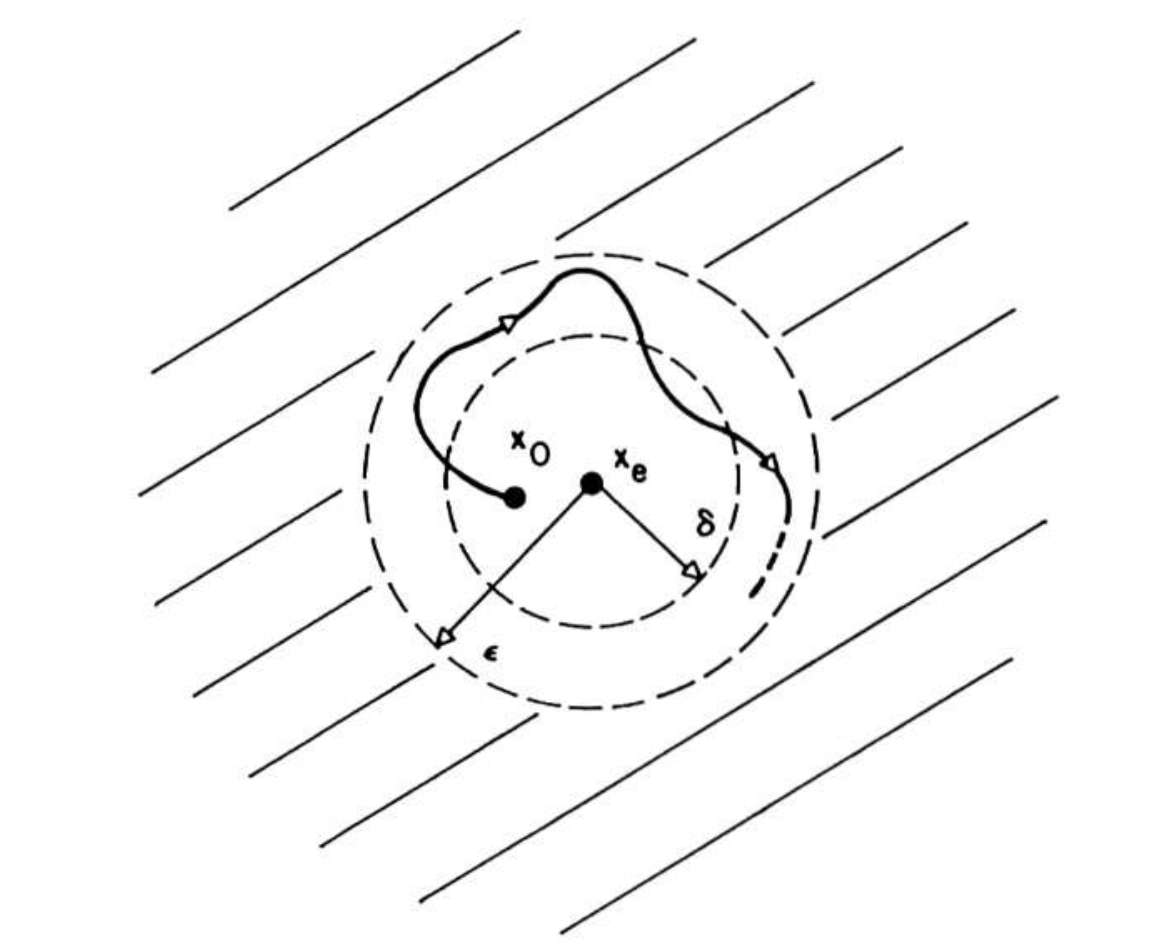Fig. 1. The basic concept of stability. Courtesy of R.E. Kalman

Put differently, the system trajectory can be kept arbitrarily close to the origin/equilibrioum if we start the trajectory sufficiently close to it. If there is stability at some initial time, $t_0$, there is stability for any other initial time $t_1$, provided that all motions are continuous in the initial state.

• Asymptotic stability: The requirement that we start sufficiently close to the origin and stay in the neighborhood of the origin is a rather limiting one in most practical engineering applications. We would want to require that our motion should return to equilibrium after any small perturbation. Thus, the classical definition of Lyapunov stability is
• an equilibrium state $x_e$ of a free dynamic system is asymptotically stable if
• it is stable; and
• every motion starting sufficiently near $x_e$ converges to $x_e$ as $t \rightarrow \infty$.
• Put differently, there is some real constant $r(t_0)>0$ and to every real number $\mu > 0$ there corresponds a real number $T(\mu, x_0, t_0)$ such that $||x_0 - x_e|| \le r(t_0)$ implies

\begin{align} ||\Phi(T; x_0, t_0)|| \le \mu \quad \forall \quad t \ge t_0 + T \nonumber \end{align}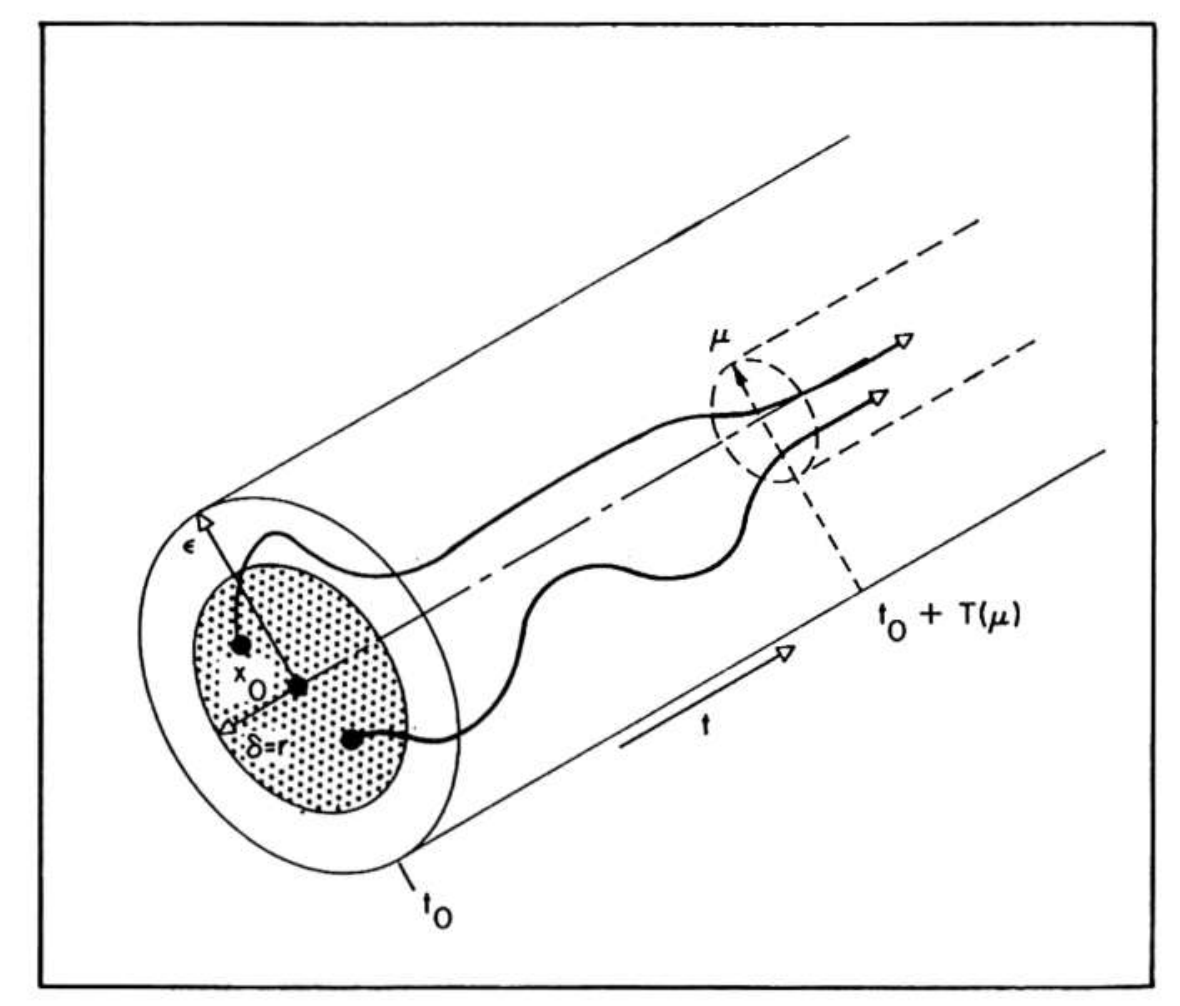Fig. 2. Definition of asymptotic stability. Courtesy of R.E. Kalman

Asymptotic stability is also a local concept since we do not know aforetime how small $r(t_0)$ should be. For motions starting at the same distance from $x_e$, none will remain at a larger distance than $\mu$ from $x$ at arbitrarily large values of time. Or to use Massera’s definition:

• An equilibrium state $x_e$ of a free dynamic system is equiasymptotically stable if
• it is stable
• every motion starting sufficiently near $x_e$ converges to $x$, as $t \rightarrow \infty$ uniformly in $x_0$

Interrelations between stability concepts

This from Kalman’s Second method of Lyapunov paper, 1960: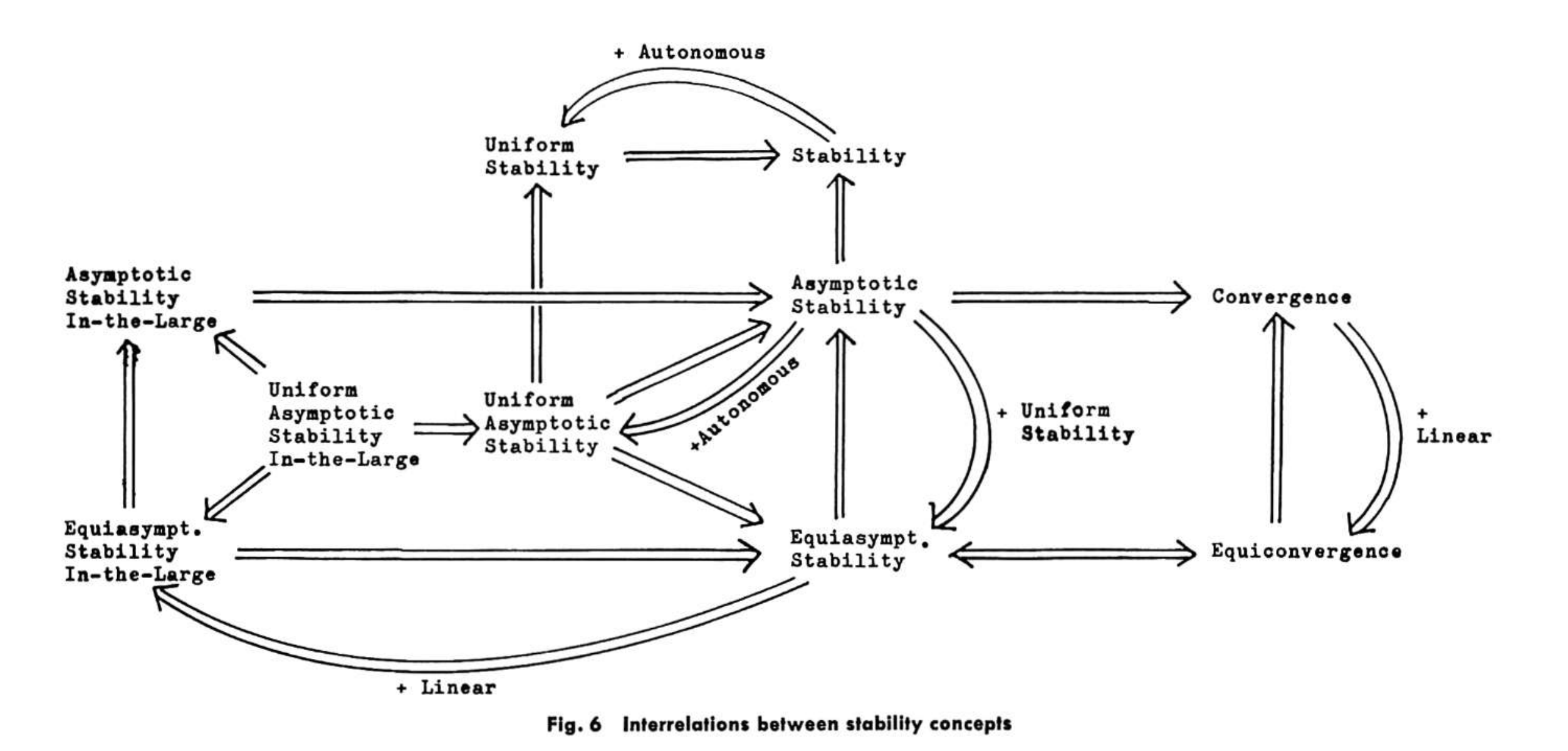Fig. 3. Interrelations between stability concepts. Courtesy of R.E. Kalman
• For linear systems, stability is independent of the distance of the initial state from $x_e$. Nicely defined as such:
• an equilibrium state $x_e$ of a free dynamic system is asymptotically (equiasymptotically) stable in the large if (i) it is stable

(ii) every motion converges to $x_e$ as $t \rightarrow \infty$, i.e., every motion converges to $x_e$, uniformluy in $x_0$ for $x_0 \le r$, where $r$ is fixed but arbitrarily large

### Control Barrier Functions

For the nonlinear control affine function,

\begin{align} \dot{\boldsymbol{x}} = \boldsymbol{f}(\boldsymbol{x}) + \boldsymbol{g}(\boldsymbol{x})\boldsymbol{u} \label{eq:cbfs::control_affine} \end{align}

where $\boldsymbol{x} \in \mathbb{R}^n$, $\boldsymbol{u} \in \mathbb{R}^m$, and $\boldsymbol{f}: \mathbb{R}^n \rightarrow \mathbb{R}^n$, and $\boldsymbol{g}: \mathbb{R}^n \rightarrow \mathbb{R}^n \times \mathbb{R}^m$ are locally Lipschitz functions, with continuity on $\mathbb{R}^n$. For a Lipschitz continuous state feedback $\boldsymbol{k}: \mathbb{R}^n \rightarrow \mathbb{R}^m$, we can write the closed-loop system dynamics as

\begin{align} \dot{\boldsymbol{x}} = \boldsymbol{f}_{cl} \triangleq \boldsymbol{f}(\boldsymbol{x}) + \boldsymbol{g}(\boldsymbol{x})\boldsymbol{k}(\boldsymbol{x}). \label{eq:cbf::closed_loop} \end{align}

whereupon the locally Lipschitz continuous property of $\boldsymbol{f}, \boldsymbol{g}$, and $\boldsymbol{k}$ implies that $\boldsymbol{f}_{cl}$ takes the local Lipzchitz continuity property. Thus, for any initial condition $\boldsymbol{x}_0: \boldsymbol{x}(0) \in \mathbb{R}^n$, we must have a maximum time interval $I(\boldsymbol{x}_0) = [0, t_{max})$ such that $\boldsymbol{x}(t)$ is the solution of \eqref{eq:cbf::closed_loop} on $I(\boldsymbol{x}(0))$. Here, $\boldsymbol{f}_{cl}$ is forward complete, $t_{max} = \infty$.

#### Safe Sets, and Invariance

Safety with CBFs are in general realized with the notion of safe sets within the state space that the system must remain in order for it to be considered safe.

We define a zero-superlevel set $\mathcal{C} \subset \mathbb{R}^n$ of a continuously differentiable function $h: \mathbb{R}^n \rightarrow \mathbb{R}$, i.e.

\begin{align} \mathcal{C} = \{ \boldsymbol{x} \in \mathbb{R}^n: h(x) \ge 0 \}, \end{align}

such that on the boundary of the superlevel set, $\partial \mathcal{C} \triangleq \{ \boldsymbol{x} \in \mathbb{R}^n: h(x) = 0 \}$; and in the interior of the $0$-superlevel set, we have $Int (\mathcal{C}) \triangleq \{ \boldsymbol{x} \in \mathbb{R}^n: h(x) > 0 \}$. It is generally assumed that $\mathcal{C}$ is nonempty (\i.e. $\mathcal{C} \neq \emptyset$) and it contains no isolated points, (i.e. $Int (\mathcal{C}) = \mathcal{C}$). $\mathcal{C}$ defines the safe set. Let us now define the concept of forward invariance and safety:

#### Forward Invariance and Safety

A set $\mathcal{C} \subset \mathbb{R}^n$ is said to be forward invariant if for every $\boldsymbol{x}_0 \in \mathcal{C}$, the solution $\boldsymbol{x}(t)$ of \eqref{eq:cbf::closed_loop} satisfies $\boldsymbol{x}(t) \subset \mathcal{C}$ for all $t \in I(\boldsymbol{x_0}$. The system in \eqref{eq:cbf::closed_loop} is said to be safe if it exists on the $0$-superlevel set $\mathcal{C}$ such that the set $\mathcal{C}$ is forward invariant.

#### Digression: Class $\mathcal{K}$ functions

Here, I give a few background definitions that will enable us to define control barrier functions adequately. This matlab script gist describes a few class-$\mathcal{K}$ functions that you may find helpful to aid the understanding.

• Class $\mathcal{K}$ Function: Supose we have a continuous function $\alpha: [0, a) \rightarrow \mathbb{R}_+$, where $a>0$, we say $\alpha$ is a class $\mathcal{K}$ ($\alpha \in \mathcal{K}$) function if $\alpha(0) = 0$ and $\alpha$ is strictly monotonically increasing.

• Class $\mathcal{K}_\infty$ Function: Supose we have a continuous function $\alpha: [0, a) \rightarrow \mathbb{R}_+, a = \infty$, we say $\alpha$ is a class $\mathcal{K}_\infty \, (\alpha \in \mathcal{K}_\infty$) function if $\alpha(0) = 0$ and $\lim_{r\rightarrow \infty}\alpha(r)=\infty$ is strictly monotonically increasing.

• Class $\mathcal{KL}$ Function: Supose we have a continuous function $\beta: [0, a) \times [0, \infty) \rightarrow [0, \infty)$, we say $\beta$ is class $\mathcal{KL}$ if for each fixed $s$, the mapping $\beta(r, s)$ belongs to class $\mathcal{K}$ with respect to $r$ and, for each fixed $r$, the mapping $\beta(r, s)$ is decreasing with respect to $s$ and $\beta(r,s)\rightarrow 0$ as $s\rightarrow\infty$.

• Extended class $\mathcal{K}$ Function: A continuous function $\alpha: (-b, a) \rightarrow \mathbb{R}$ with $a, b > 0$, belongs to the extended class $\mathcal{K}$ ($\alpha \in \mathcal{K}_e$ ) if $\alpha(0) = 0$ and $\alpha$ is strictly monotonically increasing.

• Extended class $\mathcal{K}_\infty$ Function: If $a, b = \infty, \, \lim_{r\rightarrow -\infty} \alpha(r) = -\infty$, and $\lim_{r\rightarrow \infty} \alpha(r) = \infty$, then $\alpha$ is said to belong to an extended class $\mathcal{K}_\infty (\alpha \in \mathcal{K}_{\infty, e}).$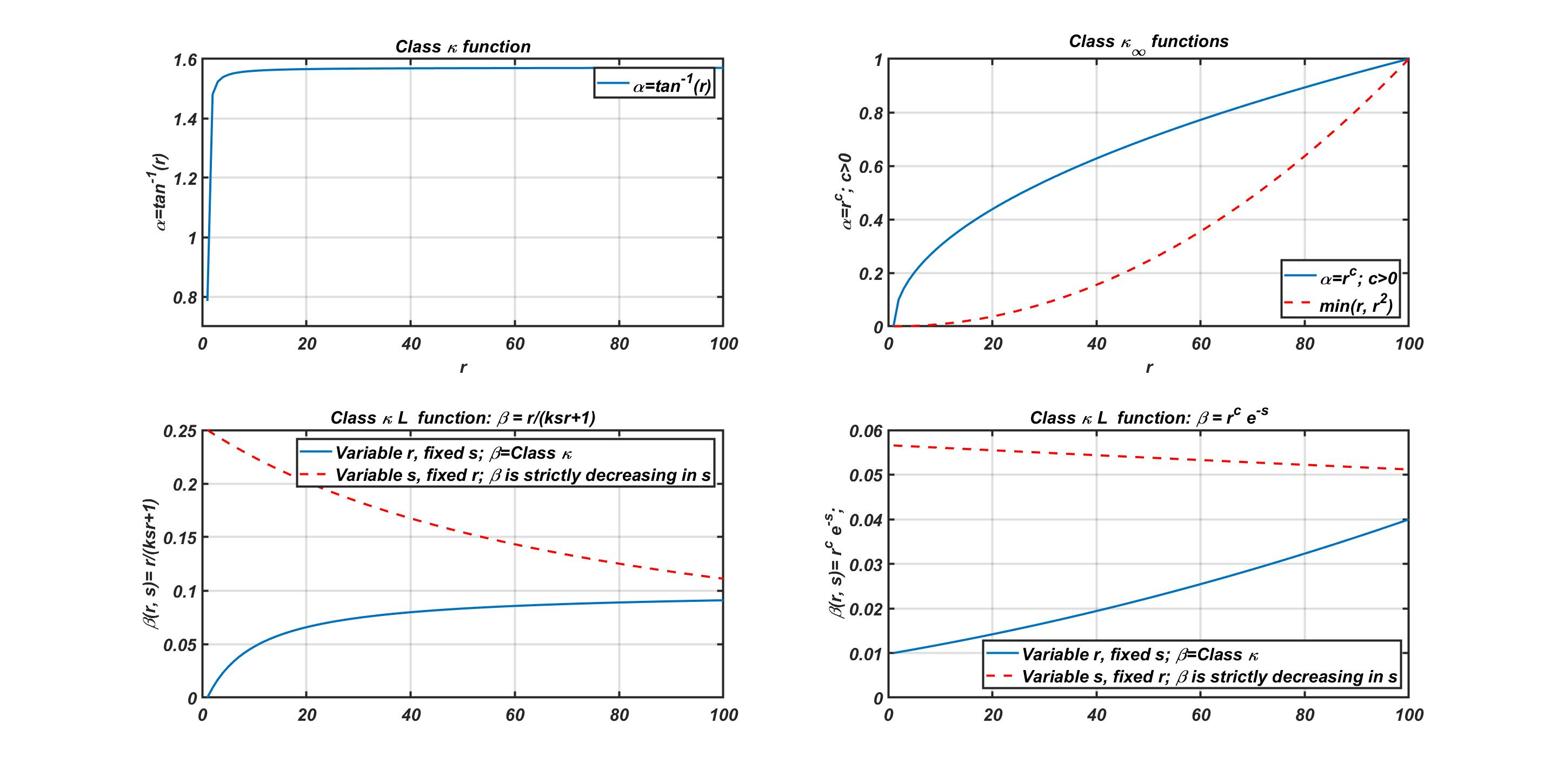Fig. 4: Class Kappa, Kappa-ell and Kappa-Infinity functions. See source script for this diagram.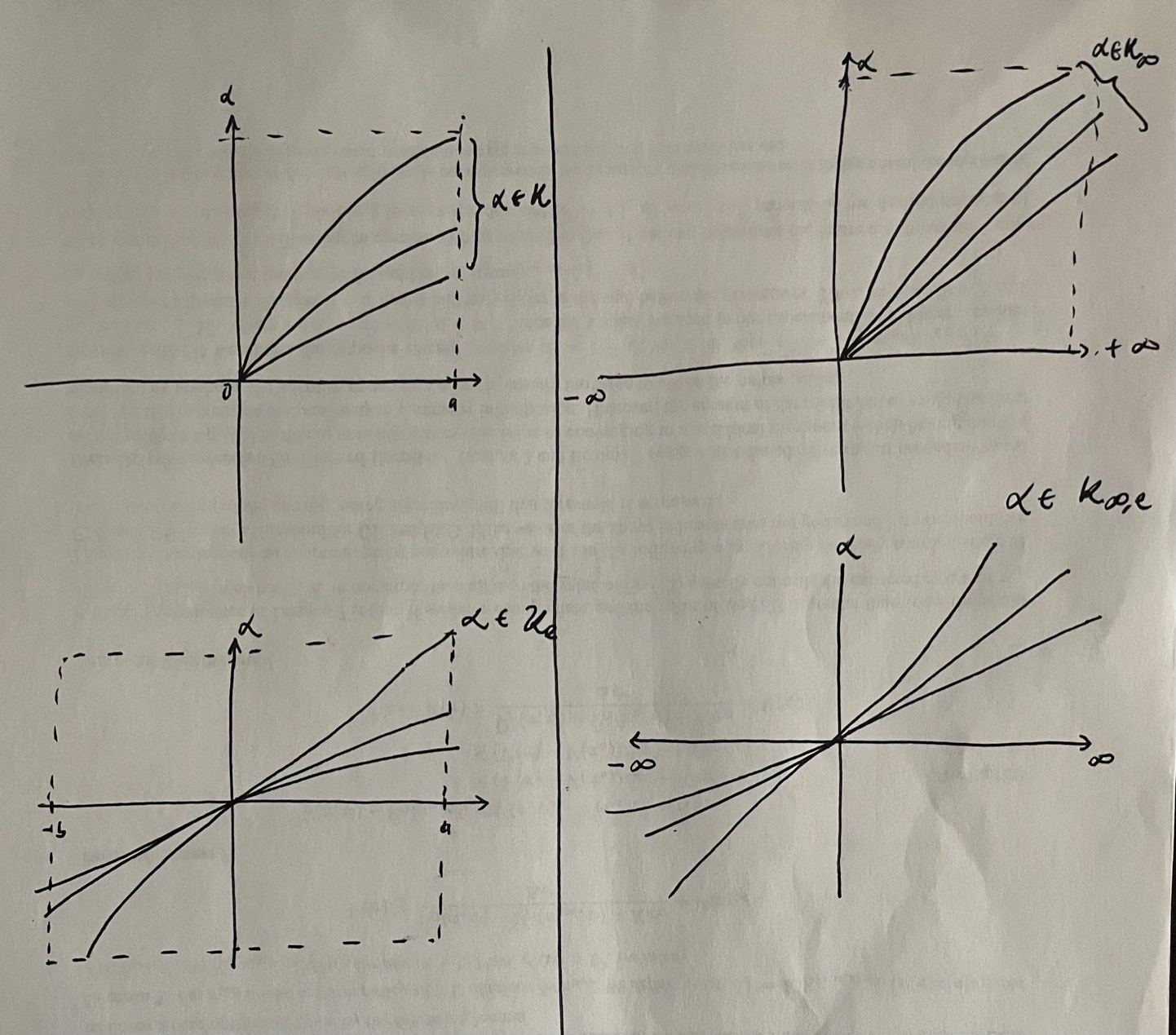Fig. 5. Naive Representation of Class Kappa and class kappa infinity extended functions. For CBFs, we are generally interested in the class-kappa infinity-extended functions.

### Control Barrier Functions: A Definition

Now that we have an understanding of the prerequisites, we can give a statement that describes CBFs.

Suppose that $\mathcal{C} \subset \mathbb{R}^n$ is a $0$-superlevel set of a continuously differentiable function $h: \mathbb{R}^n \rightarrow \mathbb{R}$ with $0$ a regular value. The function $h$ is a control barrier function for \eqref{eq:cbfs::control_affine} on the superlevel set $\mathcal{C}$ if there exists $\alpha \in \mathcal{K}_{\infty,e}$ such that for all $\boldsymbol{x} \in \mathbb{R}^n$, we have

\begin{align} \sup_{\boldsymbol{u} \in \mathbb{R}^m} \dot{h}(\boldsymbol{x}, \boldsymbol{u}) \triangleq \nabla h(\boldsymbol{x}) \boldsymbol{\dot{x}} \equiv \nabla h(\boldsymbol{x}) (\boldsymbol{f}(\boldsymbol{x}) + \boldsymbol{g}(\boldsymbol{x})) \end{align} \begin{align} &= \boldsymbol{L_f} h(\boldsymbol(x)) + \boldsymbol{L_g} h(\boldsymbol(x)) \boldsymbol{u} \ge -\alpha(h(\boldsymbol{x})) % \label{eq:cbfs::cbf-def} \end{align}

with $\boldsymbol{L_f} h(\boldsymbol(x))$ and $\boldsymbol{L_g} h(\boldsymbol(x))$ being Lie derivatives.

It follows that for a CBF $h$ for system \eqref{eq:cbfs::control_affine} and an $\alpha \in \mathcal{K}_{\infty, e}$, the point-wise set of all control values that satisfies \eqref{eq:cbfs::cbf-def} is given by,

\begin{align} K_{cbf}(\boldsymbol{x}) \triangleq \{ \boldsymbol{u} \in \mathbb{R}^m | \dot{h}(\boldsymbol{x, u}) \ge -\alpha(h(\boldsymbol{x})) \}. \end{align}

### Optimization-based Control

The question of unifying optimality in a control theoretical sense with stability guarantees such as the ones that Lyapunov analyses provide is a fascinating one. The beauty of CBFs is that we can elegantly write out our feedback control term for a control affine system dynamics such that we can create a safety-critical controller, as provided by the bound of the class-$\mathcal{K}_{\infty, e}$ in the definition of our CBF as given earlier as follows:

Oh, by the way, CBFs provide necessary and sufficient conditions on safety. I may flesh this out in a future post in the future. But for kicks, you may have a look at this paper: A. D. Ames, X. Xu, J. W. Grizzle and P. Tabuada, “Control Barrier Function Based Quadratic Programs for Safety Critical Systems,” in IEEE Transactions on Automatic Control, vol. 62, no. 8, pp. 3861-3876, Aug. 2017, doi: 10.1109/TAC.2016.2638961..

Safety-Critical Control

Given a nominal (but not necessarily safe) locally Lipschitz continuous controller $\boldsymbol{k}_d: \mathbb{R}^n \rightarrow \mathbb{R}^m$, we say controllers within the $K_{cbf}$ set is the safety-critical CBF-QP:

\begin{align} \arg \min_{\boldsymbol{u}\in \mathbb{R}^m} \frac{1}{2} ||\boldsymbol{u} - \boldsymbol{k}_d(\boldsymbol{x})||_2^2 \quad \text{such that} \,\, \dot{\boldsymbol{h}(\boldsymbol{x, u})} \ge -\alpha(h(\boldsymbol(x))). \end{align}

[To be continued]

Last updated: May 31, 2021